Courses

# Chapter Notes - Atoms and Molecules Class 9 Notes | EduRev

## Class 9 : Chapter Notes - Atoms and Molecules Class 9 Notes | EduRev

The document Chapter Notes - Atoms and Molecules Class 9 Notes | EduRev is a part of the Class 9 Course Science Class 9.
All you need of Class 9 at this link: Class 9

1. Ancient Indian and Greek philosophers have always wondered about the unknown and unseen form of matter. The idea of divisibility of matter was considered long back in India. Around 500 bc, an Indian philosopher Maharishi Kanad postulated that if we go on dividing matter (padarth), we shall get smaller and smaller particles. Ultimately, we shall come across the smallest particles, named parmanu beyond which further division will not be possible. Another Indian philosopher Pakudha Katyayama was of the opinion that various forms of matter are obtained by the combination of particles.
2. Greek philosophers Democritus and Leucippus gave similar opinion about divisibility of matter at around the same time. After a lot of experimentation, Lavoisier and Proust established the laws of chemical combination.
3. By the end of eighteenth century, scientists recognised the difference between elements and compounds and became interested in finding out how and why elements combine and what happens when they combine.
4. Law of conservation of mass: Law of conservation of mass states that mass can neither be created nor destroyed in a chemical reaction.
Activity 1. Describe an activity to illustrate the law of conservation of mass.
Materials required : Barium chloride, sodium sulphate, conical flask, cork, thread, ignition tube, weighing balance.
Procedure:
(i) Prepare separately 5% solutions of barium chloride and sodium sulphate.
(ii) Take about 10 mL of sodium sulphate in the conical flask and 10 mL of barium chloride solution in the ignition tube.
(iii) Hang the ignition tube in the flask carefully. See that the solutions do not get mixed, [see Fig. 3.1]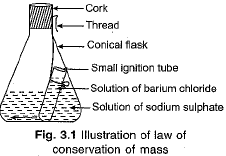(iv) Put a cork on the flask.
(v) Weigh the flask with its contents carefully.
(vi) Now shake and tilt the flask so that the solution of barium chloride mixes with the solution of sodium sulphate.
(vii) Take the weight again.
(1) We notice that a reaction takes place and a precipitate of barium sulphate is obtained.
(2) The cork is put on the mouth of the flask, so that the contents of the flask (reactants or product) do not leave the flask.
As a result of chemical change, heat is produced. Some water in the flask may be converted into vapours which might leave the flask. The cork on the mouth of the flask prevents that.
(3) We Find that the mass of the flask remains the same, before and after the reaction. In place of sodium sulphate + barium chloride, we can also take the combinations copper sulphate + sodium carbonate or lead nitrate + sodium chloride.
5. Law of constant proportion: The law states that in a chemical substance, the elements are always present in definite proportions by mass. For example, water always contains hydrogen and oxygen in the same proportion 1 : 8 by mass, whatever the source of water, from the river, or well or rainwater.
6. Dalton’s atomic theory: The postulates of Dalton’s atomic theory are:
(i) All particles are made up of very tiny particles called atoms.
(ii) Atoms are indivisible particles which cannot be created or destroyed in a chemical reaction.
(iii) Atoms of a given element are identical in mass and chemical properties.
(iv) Atoms of different elements have different mass and chemical properties.
(v) Atoms combine in the ratio of whole numbers to form compounds.
(vi) The relative number and kinds of atoms are constant in a given compound.
7. About the atom: Atoms are very small, they are smaller than anything that we can imagine or compare with. Atomic radius is measured in nanometers.
1 nm = 10-9 m
8. Dalton’s symbols of elements : Dalton’s symbols of some elements are given in Fig. 3.2. These are of historical importance now.
Nowadays IUPAC (International Union of Pure and Applied Chemistry) approves the names.
Many of the symbols are the first one or two letters of the name of the element in English. Some symbols have been taken from the names of the elements in Latin, German or Greek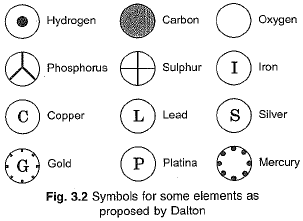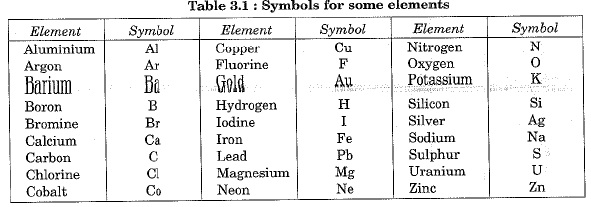9. Atomic mass: In 1961, C-12 isotope was chosen as a standard reference for measuring atomic masses. One atomic mass unit is a mass equal to exactly one-twelfth (1/12th) the mass of one atom of carbon-12. The relative atomic masses of all elements have been found with respect to an atom of carbon-12. The unit of atomic mass according to latest IUPAC recommendation is ‘u’ Earlier, it used to be a.m.u. (atomic mass unit). C-12 has been chosen as standard because we obtain nearly whole number for the atomic masses of other elements. Atomic masses of common elements are given in the table below.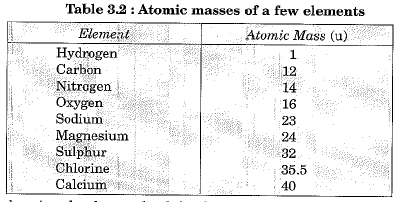10. Molecule: A molecule may be defined as the smallest particle of an element or a compound that is capable of independent existence and shows all the properties of that substance.
Molecules of elements: Molecules of elements such as helium (He), argon (Ar) etc., are made up of only one atom of that element.
Molecules of elements such as oxygen, nitrogen and chlorine, etc., are made up of two atoms o f that elements (as O2, N2, Cl2, etc.).
Molecule of ozone is comprised of three oxygen atoms (O3).
The number of atoms present in a molecule of an element is called its atomicity. Atomicities of some elements (metals and non-metals) are given in the table below: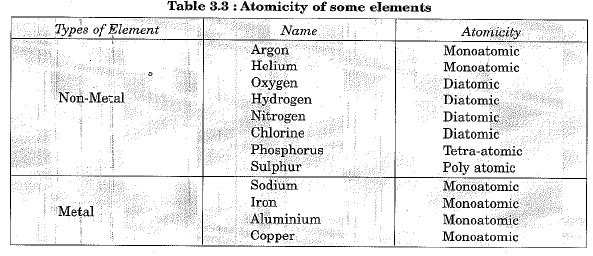Molecules of compounds: Atoms of different elements join together in definite proportions to form molecules of compounds. Some examples are given in the table below: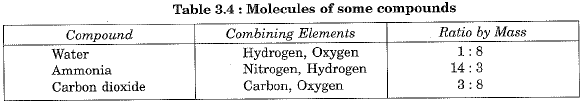11. The ratio by number of atoms for water molecule can be obtained as under: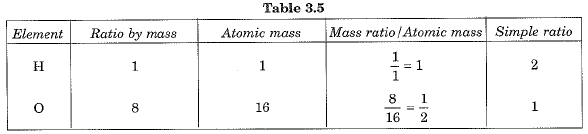12. Ions: An ion is a charged particle. It can be negatively or positively charged. Negatively charged ion is called anion while a positively charged ion is called cation. Take the case of sodium chloride. It consists of two parts, positively charged sodium ion (Na+) and negatively charged chloride ions (Cl-).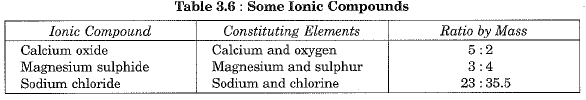13. Writing chemical formula: Chemical formula of a compound is a symbolic representation of its composition. Before we come to write chemical formula, we must know the symbols and combining capacity (valency) of elements. Valency of an atom may be thought of as hands or arms of that atom. Valencies of some simple and polyatomic ions are given in the table below: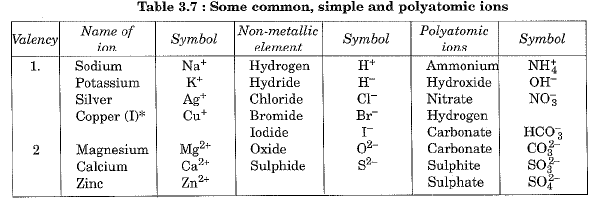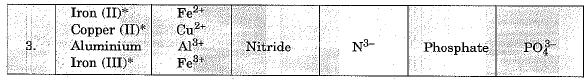Some elements show more than one valency. A Roman numeral shows their valency in a bracket.
Following rules are observed while writing a chemical formula:
(i) The valencies or charges on the ion must balance.
(ii) When a compound consists of a metal and a non-metal, the name or symbol of the metal is written first. For example, in calcium oxide (CaO), sodium chloride (NaCI), iron sulphide (FeS), copper oxide (CuO), etc., where oxygen, chlorine, sulphur are non-metals and are written on the right hand side. Whereas calcium, sodium, iron and copper are metals and are written on the left hand side.
(iii) In compounds formed with polyatomic ions, the ion is enclosed in a bracket before writing the number to indicate ratio. This is illustrated with the help of following examples: While writing chemical formulae for compounds we write the constituent elements and their valencies as shown below. Then the valencies of the combining atoms are crossed over.
(1) Formula of hydrogen chloride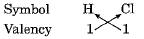Thus the formula of the compound will be or H1Cor HCl.
(2) Formula of water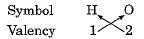The formula of the compound will be H2O1 or H20.
(3) Formula of hydrogen sulphide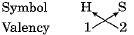The formula of the compound will be H2S1 or H2S.
(4) Formula of carbon tetrachloride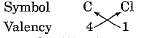The formula of the compound will be CCl4
(5) Formula of magnesium chloride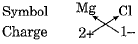Formula of magnesium chloride : MgCl2
The signs of charges (+ or -) are ignored.
(6) Formula of aluminium oxide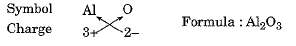(7) Formula of calcium oxide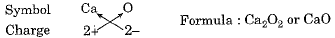(8) Formula of sodium nitrate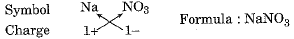(9) Formula of calcium hydroxide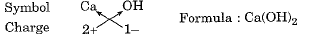(10) Formula of sodium carbonate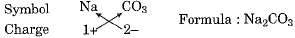(11) Formula of ammonium sulphate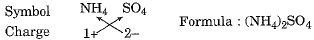14. Molecular mass: Molecular mass of a substance is the sum of atomic masses of all the atoms in a molecule of the substance. It is therefore the relative mass of a molecule expressed in atomic mass units (u). For example, molecular mass of water (H2O) is
(2 x 1) + (1 x 16) = 18 u
15. Formula unit mass: Formula unit mass of a substance is a sum of the atomic masses of all atoms in a formula unit of the compound. It is calculated in the same manner as we calculate the molecular mass. The only difference is we use the word formula unit for those substances whose constituent particles are ions. For example, sodium chloride has the formula unit NaCl. Its formula unit mass is:
(1 x 23)+ (1 x 35.5)=58.5 u
16. Mole concept: One mole of a species (atom, molecules, ions or particles) is that quantity in number having a mass equal to its atomic or molecular mass in grams. The number of particles present in 1 mole of a substance is fixed. Its value is 6.022 x 1023. This number is called Avogadro’s number or Avogadro’s constant (represented by N0).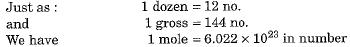Chemists need the number of atoms and molecules while carrying out reactions, and for this they need to relate the mass in grams to the number. It is done as follows:
1 mole = 6.022 x 1023 number = Relative mass in grams.
The word “mole” was introduced around 1896 by Wilhelm Ostwald who derived the term from the Latin word moles meaning a ‘heap’ or ‘pile’.

Offer running on EduRev: Apply code STAYHOME200 to get INR 200 off on our premium plan EduRev Infinity!

## Science Class 9

143 videos|224 docs|135 tests

,

,

,

,

,

,

,

,

,

,

,

,

,

,

,

,

,

,

,

,

,

;